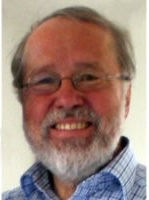Press "Enter" to skip to content

# Month: June 2018Seppo Linnainmaa (1945 — ) Inventor of automatic differentiation in 1970.

This micro post is about automatic differentiation, a programming technique allowing to compute automatically the derivative of a function from its source code. This technique, in its reverse mode, is attributed to Seppo Linnainmaa who introduced the idea in his Master thesis in 1970.

How to compute the derivative of a numerical function? If we have a symbolic mathematical expression for it, then we can use the rules of differential calculus, and this can be automated: this is known as symbolic differentiation. If the function is a black box, then we can compute numerically the differential ratio by using evaluations for very close arguments: this is known as numerical differentiation. But actually, if we have the source code of the function, one could follow the code and create an augmented code in parallel that computes the effect of each elementary operation on the derivative: this is known as automatic differentiation. Automatic differentiation can be implemented by using object programming and operators overloading. Automatic differentiation can be in trouble in the presence of conditionals.

Nowadays automatic differentiation is fashionable, partly because of the development of deep machine learning and multi-layered neural networks. For more,  take a look at the Wikipedia article. If you like the Julia programming language, you may take a look at JuliaDiff.

Last Updated on 2020-01-16Discrete two dimensional Gaussian Free Field

We have discussed in a previous post the discrete Gaussian Free Field (GFF) on the square lattice, which is the Gaussian law on say a cube $[-L,L]^d$ of $\mathbb{Z}^d$ with density proportional to

$$\mathrm{e}^{-\frac{1}{2}\sum_{i\sim j}(x_i-x_j)^2}=\mathrm{e}^{-\frac{1}{2}\langle\Delta x,x\rangle}$$

where $\Delta$ is here the discrete Laplacian on $\mathbb{Z}^d$. Integrability can be obtained by adding boundary conditions. Since this density is Gaussian, the covariance matrix is $\Delta^{-1}$, which produces a log-correlated random field if $d=2$.

The aim of this tiny post is to convince the reader that a continuous Gaussian Free Field pops up naturally in the asymptotic analysis of Coulomb gases. Recall that a Coulomb gas in dimension $d\geq1$ is the Boltzmann-Gibbs measure $P$ on $(\mathbb{R}^d)^N$ with density proportional to

$$\mathrm{e}^{-\beta H(x)}$$

where $\beta$ is a real parameter, and where

$$H(x)=\frac{1}{N}\sum_{i=1}^NV(x_i)+\frac{1}{2N^2}\sum_{i\neq j}W(x_i-x_j)$$

with $x=(x_1,\ldots,x_N)\in(\mathbb{R}^d)^N$ and

$$x\in\mathbb{R}^d\mapsto W(x)=\begin{cases}-|x|&\text{if}\ d=1\\\log\frac{1}{|x|}&\text{if}\ d=2\\\frac{1}{|x|^{d-2}}&\text{if}\ d\geq3\end{cases}.$$

The case $d=2$, $V(x)=|x|^2$, $\beta=N^2$ corresponds to the famous Ginibre ensemble of random matrix theory, for which the density of $P$ is proportional to $$\mathrm{e}^{-N\sum_{i=1}^N|x_i|^2}\prod_{i<j}|x_i-x_j|^2.$$

The quantity $H(x_1,\ldots,x_N)$ can be understood as the electrostatic energy of a configuration of $N$ electric charges in $\mathbb{R}^d$ at positions $x_1,\ldots,x_N$, subject to electrostatic repulsion via $W$ and subject to the external field generated by the potential $V$. The potential $V$ has typically a confinement effect. The function $W$, known as the Coulomb, is, up to a multiplicative constant, the fundamental solution of the Laplace equation, namely, in the sense of distributions,

$$\Delta W=-c_d\delta_0.$$

Let us simplify:  $c_d=1$. If we introduce the empirical measure $\mu_N=\frac{1}{N}\sum_{i=1}^N\delta_{x_i}$ then

$$H(x_1,\ldots,x_N)=\int V\mathrm{d}\mu_N+\frac{1}{2}\iint_{\neq}W(x-y)\mathrm{d}\mu_N(x)\mathrm{d}\mu_N(y)=\mathcal{E}_{\neq}(\mu).$$

When $\beta=\beta_N\gg N$, an outcome of refined Laplace methods know as large deviation techniques is that $\mu_N$ converges as $N\to\infty$ towards the equilibrium measure $\arg\inf\mathcal{E}$ where $\mathcal{E}$ is the asymptotic energy defined by

$$\mathcal{E}(\mu)=\int V\mathrm{d}\mu+\frac{1}{2}\int U_\mu\mathrm{d}\mu\quad\text{where}\quad U_\mu=W*\mu.$$

The quantity $U_\mu(x)$ is the potential of $\mu$ at point $x$ and $-\nabla U_\mu(x)$ is the Coulomb force generated by the configuration of charges $\mu$ at point $x$. This function is the inverse of the Laplacian in the sense of distributions

$$\Delta U_\mu=(\Delta W)*\mu=-\mu.$$

Also formally

$$\mathcal{E}(\mu)=\int V\mathrm{d}\mu+\frac{1}{2}\iint(-\Delta^{-1}\mu)\mathrm{d}\mu=\langle V,\mu\rangle+\frac{1}{2}\langle (-\Delta)^{-1}\mu,\mu\rangle$$

The right hand side is a quadratic form in $\mu$. This suggests that when $N$ gets large, the probability measure $P$, viewed as the law of the random probability measure $\mu$ on $\mathbb{R}^d$, is Gaussian with mean related to $V$ and with covariance proportional to $-\Delta$, and thus $\Delta^{-1}\mu=-U_\mu$ will be Gaussian with covariance matrix $\Delta^{-1}$: this is exactly what is known as a Gaussian Free Field (GFF). It is thus easy to guess the GFF hidden in Coulomb gases.

However making all this precise and rigorous takes pages and is technically tedious. For more details, we refer for instance to the old and new mathematical works 743526 1080535 0606663 1706.09663 1609.08088 1609.08582 on central limit theorems for the asymptotic fluctuations of Coulomb gases. More generally, at the structural level, one can retain that the natural candidate for the covariance of the fluctuation in the convergence from the Laplace method is given by the inverse of the Hessian of the energy.Voici la couverture tarte à la crème d’un livre que lit l’un de mes collègues. Le tout quantitatif des temps actuels permet en effet de s’approcher un peu plus du rêve utilitariste et capitaliste de l’optimisation universelle, pour le meilleur et pour le pire. Mais cela n’est sans doute qu’un début. À la puissance de l’informatique succédera peut-être celle de son mariage à la biologie. En ces temps de révolution, j’ai l’impression parfois d’un vide intellectuel autour du quantitatif, l’impression que les scientifiques philosophes et les philosophes des sciences occupent peu le terrain de la pensée. Mais est-ce si différent que cela du dix-neuvième siècle finalement ?

Syntax · Style · .